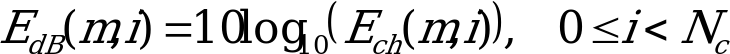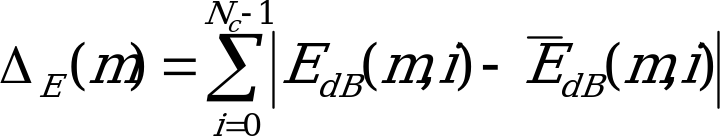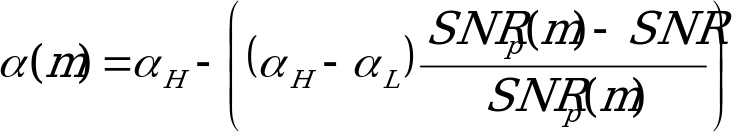## 4.3.8 Spectral Deviation Estimator

06.943GPPTSVoice Activity Detector (VAD) for Adaptive Multi Rate (AMR) speech traffic channels

The spectral deviation estimator is used as a safeguard against erroneous updates of the background noise estimate. If the spectral deviation of the input signal is too high, then the background noise estimate update may not be permitted. Calculate the estimated log power spectrum as:(4.16)

Then, calculate the estimated spectral deviation between the current power spectrum and the average long-term power spectral estimate:(4.17)

where is the average long-term power spectral estimate calculated during the previous subframe, as defined in Equation 4.20. The initial value of , however, is defined to be the estimated log power spectrum of subframe 1, or:(4.18)

The exponential windowing factor,(m), is then calculated as a function of the instantaneous frame SNR SNR and the long-term peak SNR SNRp(m), as:, (4.19)

which is then limited to.

The average long-term power spectral estimate is then updated for the next frame by:

(4.20)

where all the variables are previously defined.